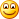Wednesday, March 27, 2013

Relearning Math

One of the problems with teaching students how to tell the computer how to do calculations is that they have to learn a new way of expressing formulas. For example one of my students tried a formula similar to this in in Excel.  =6(5+2)

Excel didn’t like it very much. It just sat there doing nothing but displaying =6(5+2) while the student complained that the computer wasn’t working. Why was it not displaying the answer 42? Those of us who have been around for a while know that there is an implied multiplication operation that is supposed to be taking place. The computer, or rather the Excel software, is not so smart and has no idea what to do though. It wants to see something more explicit like =6*(5+2)

In programming classes students, early on anyway, frequently try to write code like Balance * Rate = Interest and become confused when that gets an error. After all in math class they learn that the way formulas work is that one side of the equal sign has the same value as the other. We have to teach them that in programming the equal sign is an assignment operator that moves the value on the right to the variable on the left. Not the same thing at all. Error messages are frequently less helpful than we’d like as well.We teach math for people not for computers. I’m not sure that is wrong or even bad but it does make for more work for computer teachers. As I was thinking about this this morning I asked myself – why don’t we program the computers to understand things the way we humans understand things? Of course part of the reason is that computers are not as smart as we are. Or perhaps I should say that we have to be more literal and more exact with computers than with people. People can and do do things without being consciously aware of it. Computers can’t really do that. Let me give another example of this.

I recently asked students in my Visual Basic class to create a loop to calculate how long it took for an amount to double using compound interest. They were to create a loop that ended when the new balance was double the initial balance. About half the class tried to use code that asked if balance was equal to balance times two. This doesn’t work of course. But if they were doing this themselves in their heads or on paper or even with a calculator they would automatically (probably without conscious thought) save the initial balance and compare it with the newly calculated balance. The computer on the other hand needs to be told to save the initial balance. Stupid computer.These are the issues we struggle with when teaching students how to “do math” with computers. In programming it may be more obvious but many of the same problems exist when teaching spreadsheet or other mathematical software tools. I wonder if eventually a generation of math teachers will start teaching math differently. Maybe there is some sort of common ground that we can get to so that the way we communicate math with people and computers gets closer together. Or will there always be reteaching in some class or another?

Mike Zamansky said...

This is one of the reasons why I like using languages like scheme and NetLogo early on.

Since scheme gives us a language where everything's a function. Even arithmetic --> (+ a b) instead of a+b. Since everything's a function, everything's consistent and you don't get things like =6(5+2) - you'd get (* 6 (+ 5 2)) for the calculation and (define x (* 6 (+ 5 2))) if we want to shove it into a variable. There's no confusion between defining a variable and using = for equality testing, that is (= a b) will evaluate to true or false. It's a little wordier, but very simple.

Netlogo is similar, using let and set for assignment and = for equality testing.

Evan Pascal, with it's := made assignment easier, although it didn't solve the a*(b+c) vs a(b+c) issue.

I still see students doing the:

a+b = c

instead of the correct:

c=a+b

but less frequently than when we didn't use scheme early on.Garth said...

http://gflint.wordpress.com/2010/07/08/algebra-vs-programming-syntax-%e2%80%93-xx1-makes-no-sense/